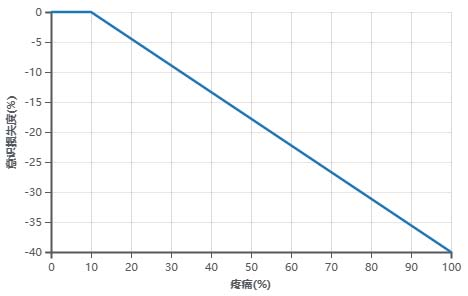## 健康系统

1. 当对应身体机能降低至0%(无)时，是否导致该单位立即死亡。
2. 功能同“意识”。
3. 某些机械体（如机械蜈蚣机械白蚁战争女皇）没有四肢，它们直接使用身节（躯干）进行移动和操作。
4. 功能同“血液过滤”，但没有免疫方面的作用（机械体不会生病）。
5. 功能同“血液循环”。

### 意识

`E(C) = E(CS) * (1 - Clamp((Pain - 0.1) * 4 / 9, 0, 0.4)) * (1 - 0.2 * △E(BP)) * (1 - 0.2 * △E(Br)) * (1 - 0.1 * △E(BF))`

• E(C) 为意识值；
• E(CS) 为提供意识的核心器官（大脑/仿生大脑）的部件效率；
• Pain 为总疼痛值；
• △E(BP) = 1 - E(BP), E(BP) 为血液循环效率；
• △E(Br) = 1 - E(Br), E(Br) 为呼吸能力效率；
• △E(BF) = 1 - E(BF), E(BF) 为血液过滤效率；
• Clamp函数的作用是把一个值限制在一个上限和下限之间，当这个值超过最小值和最大值的范围时，在最小值和最大值之间选择一个值使用。

`E(C) = E(CS) * [(Pain - 0.1) * 4 / 9] * (1 - 0.2 * △E(BP)) * (1 - 0.2 * △E(Br)) * (1 - 0.1 * △E(BF))`

### 疼痛

• 体型因素：最终疼痛值=疼痛值/体型。体型由物种和生物的成长程度决定。成年人类的体型为1.0。
• 疼痛倍率因素：最终疼痛值=疼痛值x疼痛倍率。疼痛倍率是单位通过麻醉、成瘾品、植入物等途径获得的额外乘数。

• 30点烧伤=30(伤害值)x1.875%(每点烧伤的疼痛严重度)=56.25%疼痛
• 30点割伤=30(伤害值)x1.25%(每点割伤的疼痛严重度)=37.5%疼痛

`IF Pain[%] <= 10% THEN -0% ELSE -{(Pain-0.1)/2.25}[%]`